# Relationships Between Heat and Work Chapter 10 Section

• Slides: 11Relationships Between Heat and Work Chapter 10: Section 1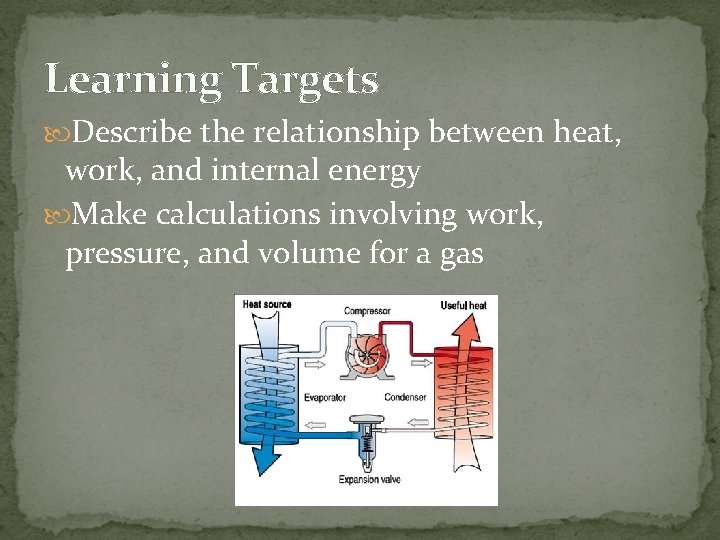Learning Targets Describe the relationship between heat, work, and internal energy Make calculations involving work, pressure, and volume for a gas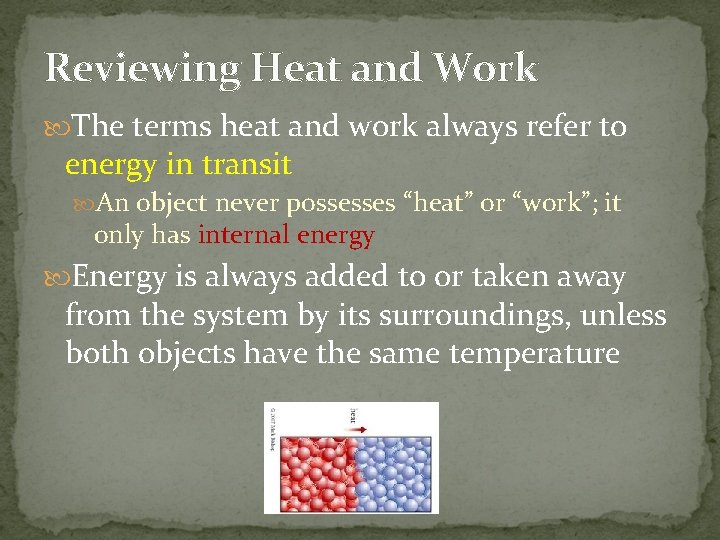Reviewing Heat and Work The terms heat and work always refer to energy in transit An object never possesses “heat” or “work”; it only has internal energy Energy is always added to or taken away from the system by its surroundings, unless both objects have the same temperature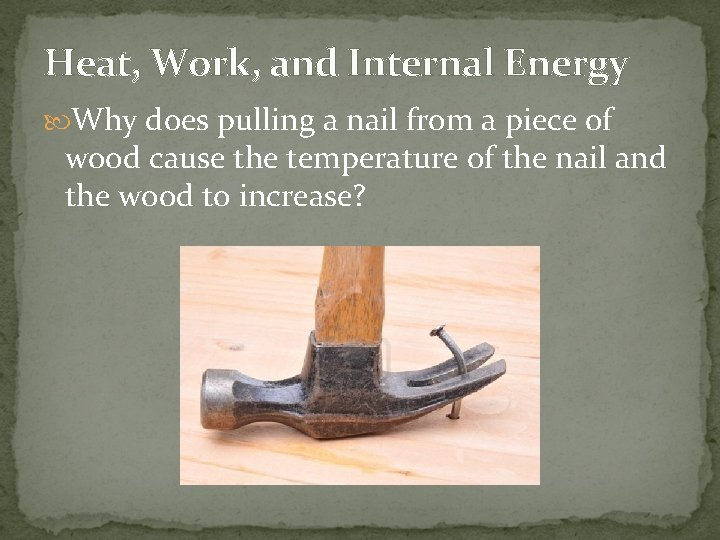Heat, Work, and Internal Energy Why does pulling a nail from a piece of wood cause the temperature of the nail and the wood to increase?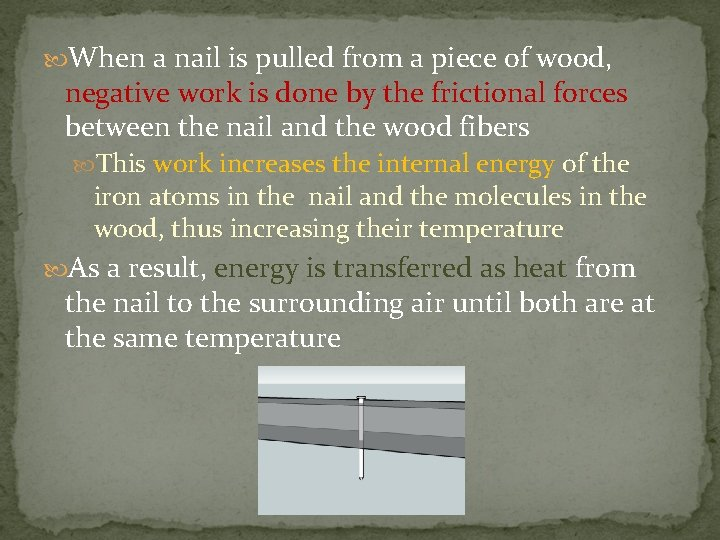When a nail is pulled from a piece of wood, negative work is done by the frictional forces between the nail and the wood fibers This work increases the internal energy of the iron atoms in the nail and the molecules in the wood, thus increasing their temperature As a result, energy is transferred as heat from the nail to the surrounding air until both are at the same temperature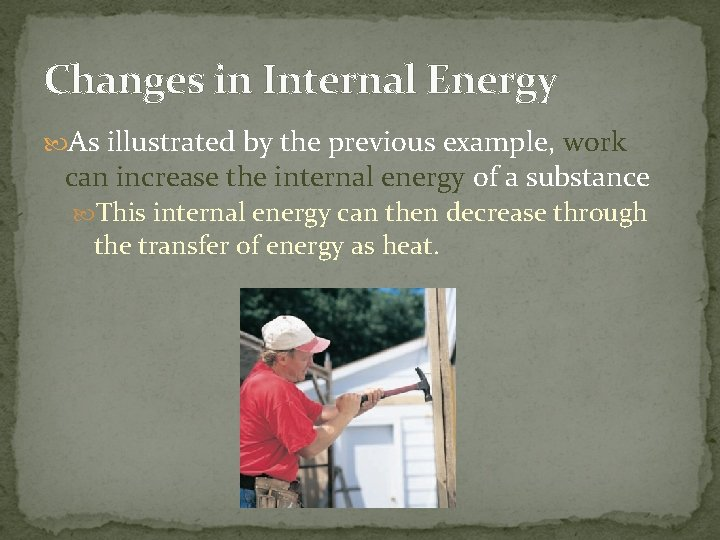Changes in Internal Energy As illustrated by the previous example, work can increase the internal energy of a substance This internal energy can then decrease through the transfer of energy as heat.On the other hand, energy can be transferred to a substance as heat, and this energy can then be used to do work Ex: Heating a flask of water with a balloon on top When the water boils and becomes steam, the steam’s volume increases and the balloon does work on the atmosphere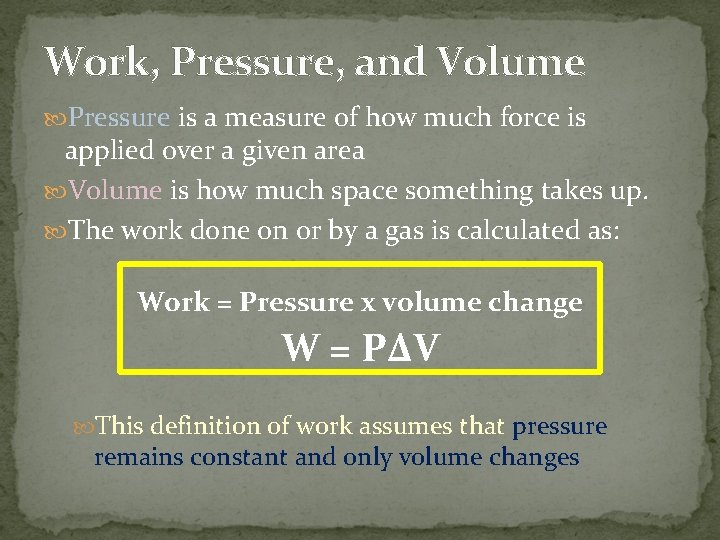Work, Pressure, and Volume Pressure is a measure of how much force is applied over a given area Volume is how much space something takes up. The work done on or by a gas is calculated as: Work = Pressure x volume change W = P∆V This definition of work assumes that pressure remains constant and only volume changes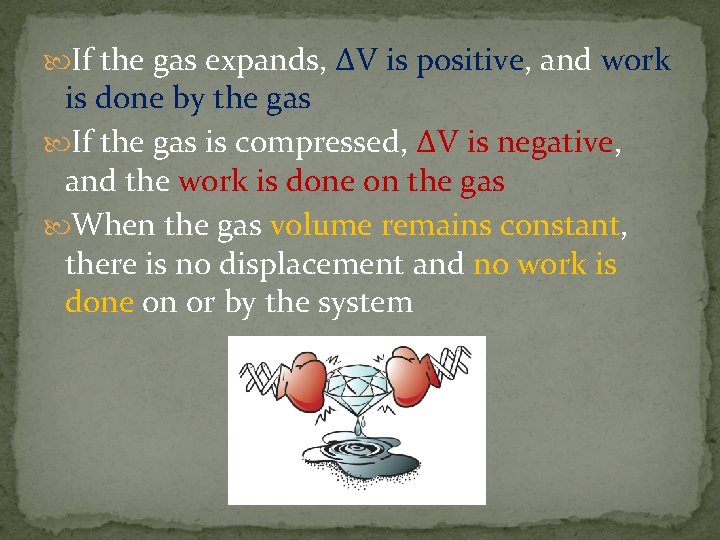If the gas expands, ∆V is positive, and work is done by the gas If the gas is compressed, ∆V is negative, and the work is done on the gas When the gas volume remains constant, there is no displacement and no work is done on or by the systemRequirements of Work Although pressure can change during a process, work is done only if the volume changes (displacement) A situation in which pressure increases and volume remains constant is comparable to one in which a force does not cause a displacementSample Problem An engine cylinder has a volume of 0. 0004 m 3. How much work can be done by a gas in a cylinder if the gas exerts a constant pressure of 7. 5 x 105 N/m 2 on the piston? 300 J